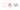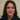# What is variable scope when we declare Javascript variables

As we learnt about ES6 variables here we need to explain more about variable scope when we are declaring them. It is essential to know where to declare which variable declaration and how to do it.

## Variable scope in Javascript

Essentially  we define scope as the current context in which values and **expressions **are “visible,” or can be referenced.

Basically we have three kind of variable declarations with different variable scope in Javascript.

As we know `var`Declares a variable, optionally initialising it to a value. Also `let`Declares a block-scoped, local variable, optionally initialising it to a value. And finally `const`Declares a block-scoped, read-only named constant.

### Variable scope of var

Firstly here is an example for “var” which each scope has been clarified in front of the variable:

``````var x = "hello world"; // scope of x: global
function foo() {
var a = 1;  // scope of a: foo
if(a > 0) {
var b = 3; // scope of b: foo
}
{
var e = 10; // scope of e: foo
}
while(a < 2) {
var d = 5; // scope of d: foo
}
}``````

Also we have global and function scope here which is not great all the time. As an example it leads to unnecessary bugs due to incomprehension of the scope.

### Variable scope of let

Secondly we have the same example with “let” and the scopes are written inside the code:

``````let x = "hello world"; // scope of x: global
function foo() {
let a = 1;  // scope of a: foo
if(a > 0) {
let b = 3; // scope of b: if statement
}
{
let e = 10; // scope of e: surrounding block
}
while(a < 2) {
let d = 5; // scope of d: while loop
}
}``````

Clearly we have statement scope, block scope, function scope and general scope. Ideally we better use let instead of var in almost all cases.

As a result let allows developers to scope variables at the block level (the nearest curly brackets).### Variable scope of const

Finally with “const” we have exactly the same result regarding to variable scope:

``````const x = "hello world"; // scope of x: global
function foo() {
const a = 1;  // scope of a: foo
if(a > 0) {
const b = 3; // scope of b: if statement
}
{
const e = 10; // scope of e: surrounding block
}
while(a < 2) {
const d = 5; // scope of d: while loop
}
}``````

Although as we know let can be mutated but const is a constant and cannot be changed once created.

However `const` does not affect whether the value of a constant itself is mutable or no. Therefor if a constant refers to an object, it will always refer to that object, but the object itself can still be changed (if it is mutable and not being freezed):

``````const obj = {};
obj.prop = 123;
console.log(obj.prop); // 123

obj = {}; // TypeError``````

### Final notes

Technically we define the scope of a variable as the region of our program in which it is defined. Traditionally, JavaScript defines only two scopes-global and local.

• Global Scope − A variable with global scope can be accessed from within any part of the JavaScript code.
• Local Scope − A variable with a local scope can be accessed from within a function or block or statement where it is declared.

As a result we better use let and var in all cases as they serve the code with all kind of scopes,

We will finish this talk with a quote of developer Aaron Frost. He put it, cheekily:  “Using LET and CONST instead of VAR will have an odd side-effect, where your code will execute at runtime just as it appears at development time.”